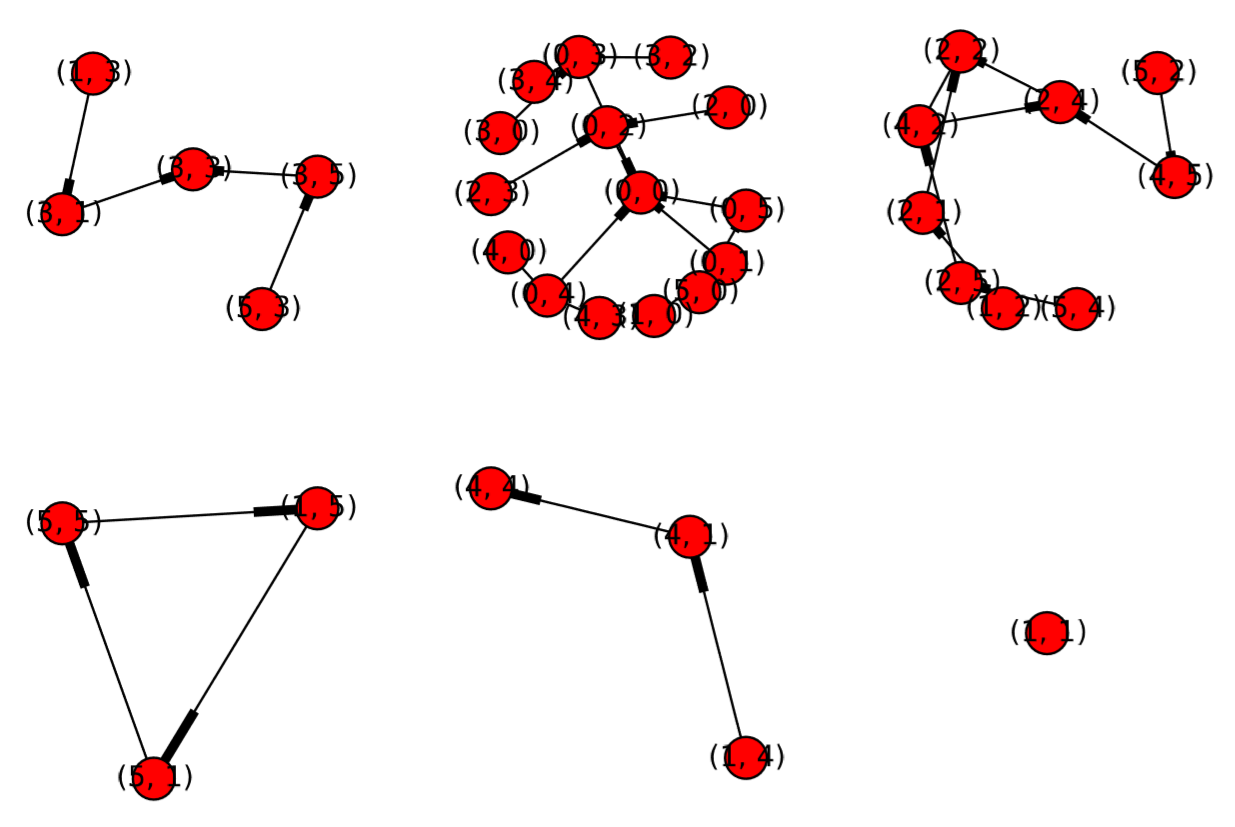$$\newcommand{\id}{\mathrm{id}}$$ $$\newcommand{\Span}{\mathrm{span}}$$ $$\newcommand{\kernel}{\mathrm{null}\,}$$ $$\newcommand{\range}{\mathrm{range}\,}$$ $$\newcommand{\RealPart}{\mathrm{Re}}$$ $$\newcommand{\ImaginaryPart}{\mathrm{Im}}$$ $$\newcommand{\Argument}{\mathrm{Arg}}$$ $$\newcommand{\norm}{\| #1 \|}$$ $$\newcommand{\inner}{\langle #1, #2 \rangle}$$ $$\newcommand{\Span}{\mathrm{span}}$$

# 5.5: Variable Rescaling of Discrete-Time Models

$$\newcommand{\vecs}{\overset { \rightharpoonup} {\mathbf{#1}} }$$

$$\newcommand{\vecd}{\overset{-\!-\!\rightharpoonup}{\vphantom{a}\smash {#1}}}$$

Aside from ﬁnding equilibrium points and visualizing phase spaces, there are many other mathematical analyses you can do to study the dynamics of discrete-time models. ButFigure $$\PageIndex{1}$$: Graph-based phase space of Eq. (5.4.1) drawn with Code 5.5.

before jumping right into such paper-and-pencil mathematical work, I would like to show you a very useful technique that can make your mathematical work much easier. It is called variable rescaling.

Variable rescaling is a technique to eliminate parameters from your model without losing generality. The basic idea is this: Variables that appear in your model represent quantities that are measured in some kind of units, but those units can be arbitrarily chosen without changing the dynamics of the system being modeled. This must be true for all scientiﬁc quantities that have physical dimensions—switching from inches to centimeters should not cause any change in how physics works! This means that you have the freedom to choose any convenient unit for each variable, some of which may simplify your model equations.

Let’s see how variable rescaling works with an example. Here is the logistic growth model we discussed before:

$x_{t} = x_{t-1} +rx_{t-1} \left(1-\dfrac{x_{t-1}}{K}\right) \label{(5.20)}$

There is only one variable in this model, $$x$$, so there is only one unit we can change, i.e., the unit of the population counts. The ﬁrst step of variable rescaling is to replace each of the variables with a new notation made of a non-zero constant and a new state variable, like this:

$x\rightarrow {\alpha}{x}' \label{(5.21)}$

With this replacement, Equation \ref{5.20} becomes

${\alpha{x}_{t}}' =\alpha{x}'_{t-1} +r\alpha{x}'_{t-1} \left(1-\dfrac{\alpha{x}'_{t-1}}{K}\right). \label{(5.22)}$

The second step is to simplify the equation and then ﬁnd a “convenient” choice for the constant that will make your equation simpler. This is a rather open-ended process with many different directions to go, so you will need to do some explorations to ﬁnd out what kind of unit choices make your model simplest. For the logistic growth model, Equation \ref{5.22} can be further simpliﬁed, for example, like

\begin{align} {x}'_{t} &={x}'_{t-1} +r{x}'_{t-1} \left(1-\dfrac{\alpha{x}'_{t-1}}{K}\right) \label{(5.23)} \\[4pt] &= {x}'_{t-1}\left(1+r\left(1-\dfrac{\alpha{x}'_{t-1}}{K}\right)\right) \label{(5.24)} \\[4pt] &= {x}'_{t-1} \left(1+r-\dfrac{r\alpha{x}'_{t-1}}{K} \right) \label{(5.25)} \\[4pt] &= (1+r){x}'_{t-1} \left(1-\dfrac{r\alpha{x}'_{t-1}}{K(1+r)}\right). \label{(5.26)} \end{align}

Here, the most convenient choice of $$α$$ would be$$α = \dfrac{K(1 + r)}{r}\label{(5.26B)}$$with which Equation \ref{5.26} becomes

${x}'_{t}=(1+r){x}'_{t-1}(1-{x}'_{t-1}). \label{(5.27)}$

Furthermore, you can always deﬁne new parameters to make equations even simpler. Here, you can deﬁne a new parameter $${r}' = 1+r$$, with which you obtain the following ﬁnal equation:

${x}'_{t} ={r}'{x}'_{t-1}(1-{x}'_{t-1}) \label{(5.28)}$

Note that this is not the only way of rescaling variables; there are other ways to simplify the model. Nonetheless, you might be surprised to see how simple the model can become. The dynamics of the rescaled model are still exactly the same as before, i.e., the original model and the rescaled model have the same mathematical properties. We can learn a few more things from this result.

• First, Equation \ref{5.26B} tells us what is the meaningful unit for you to use in measuring the population in this context.
• Second, even though the original model appeared to have two parameters ($$r$$ and $$K$$), this model essentially has only one parameter, $${r}'$$, so exploring values of $${r}'$$ should give you all possible dynamics of the model (i.e., there is no need to explore in the $$r-K$$ parameter space).

In general, if your model has $$k$$ variables, you may be able to eliminate up to $$k$$ parameters from the model by variable rescaling (but not always). By the way, this simpliﬁed version of the logistic growth model obtained above,

$x_{t} =rx_{t-1}(1-x_{t-1}), \label{(5.29)}$

has a designated name; it is called the logistic map and is arguably the most extensively studied 1-D nonlinear iterative map. This will be discussed in more detail in Chapter 8. Here is a summary of variable rescaling:

How-TO: Rescaling

You should try variable rescaling to eliminate as many parameters as possible from your model before conducting a mathematical analysis. You may be able to eliminate as many parameters as the variables in your model. To rescale variables, do the following:

1. Replace all variables with a non-zero constant times a new variable.
2. Simplify the model equations.
3. Find “convenient” choices for the constants that will make your equations as simple as possible.
4. Deﬁne new parameters, as needed, to make the equations even simpler.

Exercise $$\PageIndex{1}$$

Simplify the following difference equation by variable rescaling:

$x_{t} =\dfrac{a}{x_{t-1}+b} \label{(5.30)}$

Exercise $$\PageIndex{2}$$

Simplify the following two-dimensional predator-prey difference equation model by variable rescaling:

$x_{t} =x_{t-1}+rx_{t-1} \left(1-\dfrac{x_{t-1}}{K}\right)- \left(1-\dfrac{1}{by_{t-1} +1}\right) x_{t-1} \label{(5.31)}$

$y_{t} =y_{t-1} -dy_{t-1} +cx_{t-1}y_{t-1} \label{(5.32)}$